Analysis WebNotes
arrow_back arrow_forward

# Class Contents

## Limits of functions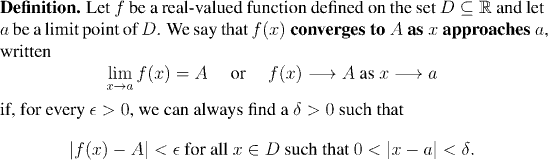Example: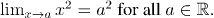Example: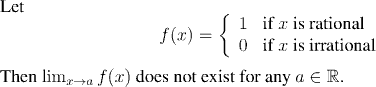Example: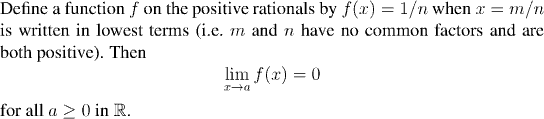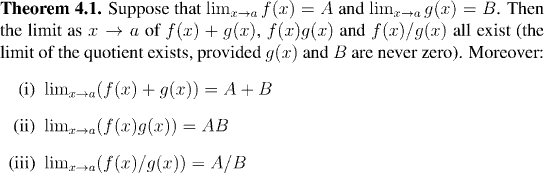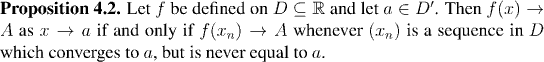Note that we can use Proposition 4.2, together with our results on the arithmetic of convergent sequences ( Theorem 3.9) to prove Theorem 4.1 quickly. If (x_n) converges to a in D but is never equal to a, then f(x_n)+g(x_n), f(x_n)g(x_n), and f(x_n)/g(x_n) converge to A+B, AB, and A/B respectively, by Theorem 3.9, and one half of Proposition 4.2.

But then the other direction in Proposition 4.2 shows that, since (x_n) was an arbitrary sequence, f(x)+g(x), f(x)g(x), and f(x)/g(x) converge to the same limits.Proof. Use Proposition 4.2 and Lemma 3.3.

Example:

We can reprove an earlier example much more easily now:Simply take a sequence p_n of rationals converging to a (without ever reaching it) and a sequence x_n of irrationals converging to a without ever reaching it. Then f(p_n)=1 and f(x_n)=0 for all n, and so the sequences cannot converge to a common limit. It follows from Proposition 4.2 that the limit of the function does not exist.